International Tables for Crystallography (2006). Vol. B, ch. 2.5, pp. 276-345   | 1 | 2 | https://doi.org/10.1107/97809553602060000558

Contents

• 2.5. Electron diffraction and electron microscopy in structure determination  (pp. 276-345)
• 2.5.1. Foreword  (pp. 276-277) | html | pdf |
• 2.5.2. Electron diffraction and electron microscopy  (pp. 277-285) | html | pdf |
• 2.5.2.1. Introduction  (pp. 277-278) | html | pdf |
• 2.5.2.2. The interactions of electrons with matter  (pp. 278-279) | html | pdf |
• 2.5.2.3. Recommended sign conventions  (pp. 279-280) | html | pdf |
• 2.5.2.4. Scattering of electrons by crystals; approximations  (pp. 280-281) | html | pdf |
• 2.5.2.5. Kinematical diffraction formulae  (pp. 281-282) | html | pdf |
• 2.5.2.6. Imaging with electrons  (pp. 282-283) | html | pdf |
• 2.5.2.7. Imaging of very thin and weakly scattering objects  (pp. 283-284) | html | pdf |
• 2.5.2.8. Crystal structure imaging  (p. 284) | html | pdf |
• 2.5.2.9. Image resolution  (pp. 284-285) | html | pdf |
• 2.5.2.10. Electron diffraction in electron microscopes  (p. 285) | html | pdf |
• 2.5.3. Space-group determination by convergent-beam electron diffraction   (pp. 285-306) | html | pdf |
• 2.5.3.1. Introduction  (pp. 285-286) | html | pdf |
• 2.5.3.1.1. CBED  (pp. 285-286) | html | pdf |
• 2.5.3.1.2. Zone-axis patterns from CBED  (p. 286) | html | pdf |
• 2.5.3.2. Background theory and analytical approach  (pp. 286-288) | html | pdf |
• 2.5.3.2.1. Direct and reciprocity symmetries: types I and II  (pp. 286-287) | html | pdf |
• 2.5.3.2.2. Reciprocity and Friedel's law  (p. 287) | html | pdf |
• 2.5.3.2.3. In-disc symmetries  (pp. 287-288) | html | pdf |
• 2.5.3.2.4. Zero-layer absences  (p. 288) | html | pdf |
• 2.5.3.3. Pattern observation of individual symmetry elements  (pp. 288-289) | html | pdf |
• 2.5.3.4. Auxiliary tables  (pp. 289-291) | html | pdf |
• 2.5.3.5. Space-group analyses of single crystals; experimental procedure and published examples  (pp. 291-292) | html | pdf |
• 2.5.3.5.1. Stages of procedure  (pp. 291-292) | html | pdf |
• 2.5.3.5.2. Examples  (p. 292) | html | pdf |
• 2.5.3.6. Use of CBED in study of crystal defects, twins and non-classical crystallography  (pp. 292-295) | html | pdf |
• 2.5.3.7. Present limitations and general conclusions  (p. 295) | html | pdf |
• 2.5.3.8. Computer programs available  (pp. 295-306) | html | pdf |
• 2.5.4. Electron-diffraction structure analysis (EDSA)  (pp. 306-310) | html | pdf |
• 2.5.4.1. Introduction  (p. 306) | html | pdf |
• 2.5.4.2. The geometry of ED patterns  (pp. 306-308) | html | pdf |
• 2.5.4.3. Intensities of diffraction beams  (pp. 308-309) | html | pdf |
• 2.5.4.4. Structure analysis  (pp. 309-310) | html | pdf |
• 2.5.5. Image reconstruction   (pp. 310-315) | html | pdf |
• 2.5.5.1. Introduction  (pp. 310-311) | html | pdf |
• 2.5.5.2. Thin weak phase objects at optimal defocus  (pp. 311-312) | html | pdf |
• 2.5.5.3. An account of absorption  (p. 312) | html | pdf |
• 2.5.5.4. Thick crystals  (p. 312) | html | pdf |
• 2.5.5.5. Image enhancement  (pp. 313-315) | html | pdf |
• 2.5.6. Three-dimensional reconstruction   (pp. 316-320) | html | pdf |
• 2.5.6.1. The object and its projection  (pp. 315-316) | html | pdf |
• 2.5.6.2. Orthoaxial projection  (pp. 316-317) | html | pdf |
• 2.5.6.3. Discretization  (p. 317) | html | pdf |
• 2.5.6.4. Methods of direct reconstruction  (p. 317) | html | pdf |
• 2.5.6.5. The method of back-projection  (p. 318) | html | pdf |
• 2.5.6.6. The algebraic and iteration methods  (p. 318) | html | pdf |
• 2.5.6.7. Reconstruction using Fourier transformation  (pp. 318-319) | html | pdf |
• 2.5.6.8. Three-dimensional reconstruction in the general case  (pp. 319-320) | html | pdf |
• 2.5.7. Direct phase determination in electron crystallography  (pp. 320-326) | html | pdf |
• 2.5.7.1. Problems with traditional' phasing techniques  (pp. 320-321) | html | pdf |
• 2.5.7.2. Direct phase determination from electron micrographs  (pp. 321-322) | html | pdf |
• 2.5.7.3. Probabilistic estimate of phase invariant sums  (pp. 322-323) | html | pdf |
• 2.5.7.4. The tangent formula  (pp. 323-324) | html | pdf |
• 2.5.7.5. Density modification  (p. 324) | html | pdf |
• 2.5.7.6. Convolution techniques  (p. 324) | html | pdf |
• 2.5.7.7. Maximum entropy and likelihood  (p. 325) | html | pdf |
• 2.5.7.8. Influence of multiple scattering on direct electron crystallographic structure analysis  (pp. 325-326) | html | pdf |
• References | html | pdf |
• Figures
• Fig. 2.5.2.1. The variation with accelerating voltage of electrons of ( a ) the wavelength, λ and ( b ) the quantity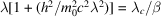(p. 279) | html | pdf |
• Fig. 2.5.2.2. Diagram representing the critical components of a conventional transmission electron microscope (TEM) and a scanning transmission electron microscope (STEM)  (p. 282) | html | pdf |
• Fig. 2.5.2.3. The functions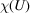, the phase factor for the transfer function of a lens given by equation (2.5.2.33), and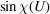for the Scherzer optimum defocus condition  (p. 283) | html | pdf |
• Fig. 2.5.3.1. Vector diagram in semi-reciprocal space, using Ewald-sphere constructions to show the incident', reciprocity' and reciprocity × centrosymmetry' sets of vectors  (p. 287) | html | pdf |
• Fig. 2.5.3.2. Diagrammatic representation of a CBED disc with symmetry lines m , m R (alternate labels G , S ) and the central point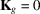(p. 287) | html | pdf |
• Fig. 2.5.3.3. Diagrammatic representation of the influence of non-symmorphic elements  (p. 289) | html | pdf |
• Fig. 2.5.3.4. ( a ) Zone-axis patterns from hexagonal structures β -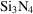(left) and β -GaS (right) together with the appropriate planar figures for point symmetries 6 and 6 mm , respectively  (p. 293) | html | pdf |
• Fig. 2.5.3.5. Zone-axis patterns from cubic structures gahnite (left) (Ishizuka & Taftø, 1982) and χ -phase precipitate (right) (Steeds & Evans, 1980)  (p. 294) | html | pdf |
• Fig. 2.5.3.6. ( a ) CBED pattern from the exact  (cyclic) zone-axis orientation of FeS 2   (p. 294) | html | pdf |
• Fig. 2.5.4.1. Ewald spheres in reciprocal space  (p. 306) | html | pdf |
• Fig. 2.5.4.2. Triclinic reciprocal lattice  (p. 307) | html | pdf |
• Fig. 2.5.4.3. Formation of ellipses on an electron-diffraction pattern from an oblique texture  (p. 307) | html | pdf |
• Fig. 2.5.5.1. The χ function and two components of the Scherzer phase function sinand cos(p. 311) | html | pdf |
• Fig. 2.5.5.2. ( a ) Diagram of an optical diffractometer  (p. 314) | html | pdf |
• Fig. 2.5.6.1. A three-dimensional object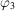and its two-dimensional projection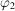(p. 316) | html | pdf |
• Fig. 2.5.6.2. The projection sphere and projectionofalong τ onto the plane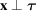(p. 316) | html | pdf |
• Fig. 2.5.6.3. Orthoaxial projection  (p. 317) | html | pdf |
• Fig. 2.5.6.4. Discretization and oblique projection  (p. 317) | html | pdf |
• Fig. 2.5.6.5. ( a ) Formation of a projection function; ( b ) superposition of these functions  (p. 318) | html | pdf |
• Fig. 2.5.6.6. Relative position of the particle and planes of projection  (p. 320) | html | pdf |
• Fig. 2.5.6.7. Section of a three-dimensional Fourier transform of the density of the particles, corresponding to plane projections of this density  (p. 320) | html | pdf |
• Fig. 2.5.6.8. Plane projections of a three-dimensional body  (p. 320) | html | pdf |
• Fig. 2.5.7.1. Potential map for diketopiperazine  (p. 323) | html | pdf |
• Tables
• Table 2.5.2.1. Standard crystallographic and alternative crystallographic sign conventions for electron diffraction  (p. 280) | html | pdf |
• Table 2.5.3.1. Listing of the symmetry elements relating to CBED patterns under the classifications of vertical' (I), horizontal' (II) and combined or roto-inversionary axes  (p. 286) | html | pdf |
• Table 2.5.3.2. Diagrammatic illustrations of the actions of five types of symmetry elements (given in the last column in Volume A diagrammatic symbols) on an asymmetric pattern component, in relation to the centre of the pattern at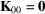, shown as ⊕', or in relation to the centre of a diffraction order at, shown as +'  (p. 288) | html | pdf |
• Table 2.5.3.3. Diffraction point-group tables, giving whole-pattern and central-beam pattern symmetries in terms of BESR diffraction-group symbols and diperiodic group symbols  (p. 290) | html | pdf |
• Table 2.5.3.4. Tabulation of principal-axis CBED pattern symmetries against relevant space groups given as numbers  (pp. 296-297) | html | pdf |
• Table 2.5.3.5. Conditions for observation of GS bands for the 137 space groups exhibiting these extinctions  (pp. 298-305) | html | pdf |